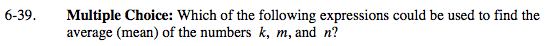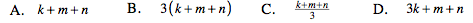### Home > MC2 > Chapter 6 > Lesson 6.1.4 > Problem6-39

6-39.The average of a set of numbers is the sum of the values divided by the number of values in the set.

Which of the above choices best represents the average? Do any choices include a
summation of the numbers k, m, and n and also divide by this number of values?

$\frac{k+m+n}{3}$